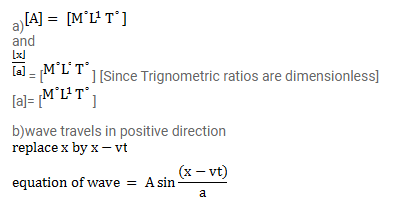# A wave pulse is travelling on a string with a speed v towards

Question:

A wave pulse is travelling on a string with a speed $v$ towards the positive $x$-axis. The shape of the string at $t=0$ is given by $\mathrm{g}(\mathrm{x})=\mathrm{A} \sin (\mathrm{x} / \mathrm{a})$, where $\mathrm{A}$ and a are constants.

(a) What are the dimensions of A and a?

(b) Write the equation of the wave for a general time $t$, if the wave of the speed is $t$.

Solution: